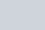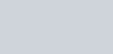•
•
•
•
•
•
•
•

# TIME DILATION: BASIC CONCEPTS

Today we are going to talk about a very interesting phenomenon, which has come into existence with Einstein’s special theory of relativity.

We are not talking about any other things, we are only talking about time dilation.In the special theory of relativity Albert Einstein shows that time is a relative quantity. According to Einstein the flow of time is the same as the flow of the river, we know that sometimes a river flows fast and sometimes this river flows slower. Exactly the same as the river, time also flows sometimes fast and sometimes slow.

But when does time flow slower and faster? This is the question. In the form of an answer, its one explanation is in the special theory of relativity, and one explanation is in the general theory of relativity.

According to the Special theory of relativity (STR), slowing and fasting of time only depend on the speed of the object.If speed is very large then time becomes very slow or you can say very small. And if speed is very small then time becomes fast or you can say it becomes large. The magnitude of dilated time is given as follows:Where t is dilated time, t0 is rest time, v is traveling velocity, c is the speed of light.

Let’s understand this statement by a thought Imagination.

Imagine two clocks, one of them is in the rocket and one is on the earth at rest. This rocket is very powerful and can fly at the speed of light. If the rocket starts flying with the speed of light then due to having maximum constant speed (Speed of light).then time in the rocket will be nearly stopped. When the rocket stopped at his destination then the people in the rocket feel that time doesn’t change so far for him. But the clock which is on the earth, time change to this for a large extent, because this clock is at rest.

But, what is time dilatation?
We will talk about it after giving the second explanation on the basic general theory of relativity.

According to this theory… slowing and fasting of time depends on the mass of the object or we can say its gravitational potential.
Take a two clock put one clock on the earth and put another clock on the very massive objects that are the black hole. Then it is noticed that the clock which is nearer or in the black hole or on any massive objects, time passes slowly for that clock. But that clock which is on the earth, time passes fastly for that. But why does time passes slowly near supermassive objects? i.e the black hole.

Let’s find out the answer to this question…

According to the general theory of relativity, we know that large masses of objects create large distortion in space-time. This large distortion gives the large space-time slope, and this large slope gives a very high acceleration due to free-falling, or simply says the acceleration due to gravity.

And sometimes this acceleration is about to speed in light, depending on the massiveness of the black hole. this is the reason why light can’t be escaped from any black hole.? but if when an object enters the slope of a black hole then he starts moving around it (revolution) with the approx the speed of light, along with the accretion disk.The motion of an object around the black hole Image credit: National Geographic channel

If it is moving around the black hole with the approx of the speed of light, then we know that time becomes slow for that object, we have seen this in the special theory of relativity. Now it’s time to give a definition to the term time dilation.

Time dilation is nothing, but it is only the measurement of the difference of the elapsed time between the two clocks, either the two clocks are in relative motion to each other or the two clocks located near the two different objects masses. (In which the first object is very massive and the second object is less massive than the first). We have discussed this definition above in full length. Time dilation gives birth to the new concept that is time travel.

•
•
•
•
•
•
•
•

Very nice